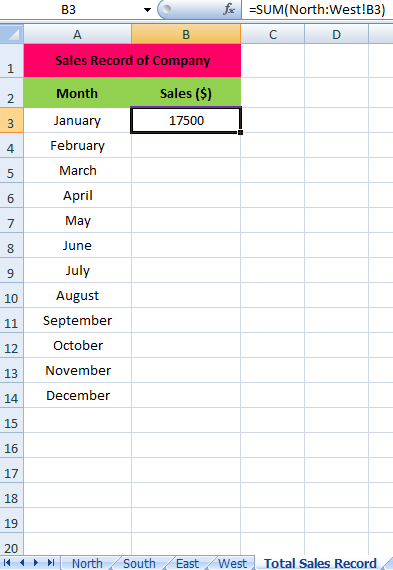### 3 D Reference in MS Excel

3D Reference in MS Excel
3D reference is one of the MS Excel greatest cell reference feature.
A 3D reference in MS Excel refers to the same cell or range of cells on multiple worksheets. By applying 3D Reference, you can calculate data across several worksheets with the same structure.
How to Create 3D Reference in MS Excel
Let's learn with Example
Below is the Example of worksheet containing Zones North, East, West and South and showing company's Total Sales Record.
>> On the Total Sales Record sheet, select cell B3 and Use the 3D Reference as following:
B3 equals =SUM(North:West!B3)Copy & Paste or Drag the Formula till Cell B14.
If you add any worksheet between North and West, the worksheet will be automatically included in the formula in cell B3.
Result